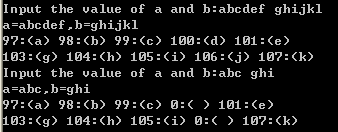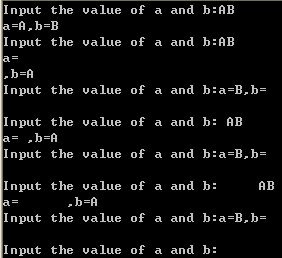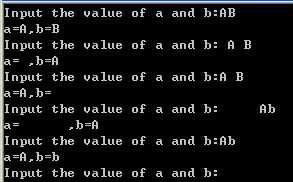# c语言中scanf函数与空格回车

1、scanf的返回值

scanf通常返回的是成功赋值(从标准输入设备赋值到参数列表所指定的内存区域)的数据项数，如果出错或是遇到end of file（注意，如果想从键盘输入EOF，在windows的DOS窗口用Ctrl+Z 或F6；在UNIX系统上，用CTRL+D。），则返回EOF，比如：

scanf("%d%d", &x, &y); scanf函数仅在每一个数据域均有数据，并按回车后结束

2、scanf的处理机制

scanf以删除的方式从缓冲区读入数据(来自标准输入设备的数据存储在缓冲区)，也就是说，scanf从缓冲区读入一个数据项，该数据项在缓冲区中就被清除掉了。而如果scanf需要读取一个数据项，发现缓冲区当前是空的，那么程序就会在scanf代码处阻塞，等待用户输入，scanf函数接收到相应的数据项之后，在缓冲区中将这一数据项清除，scanf函数返回，程序继续执行。

3、scanf对不同类型输入的处理方式

3.1 整数%d

1. Code:
2. #include<stdio.h>
3. int main()
4. {
5.     int a,b;
6.     printf("Input the value of a and b:");
7.     while(scanf("%d%d",&a,&b)!=EOF)
8.     {
9.         printf("a=%d,b=%d\n",a,b);
10.         printf("Input the value of a and b:");
11.     }
12.     return 0;
13. }
14. Output:
15. Input the value of a and b:123 456
16. a=123,b=456
17. Input the value of a and b:123  456
18. a=123,b=456
19. Input the value of a and b:123
20. 456
21. a=123,b=456
22. Input the value of a and b:
23. 123 456
24. a=123,b=456
25. Input the value of a and b:     123 456
26. a=123,b=456
27. Input the value of a and b: 123 456
28. a=123,b=456
29. Input the value of a and b:^Z
30. Press any key to continue
Code:
#include<stdio.h>
int main()
{
int a,b;
printf("Input the value of a and b:");
while(scanf("%d%d",&a,&b)!=EOF)
{
printf("a=%d,b=%d\n",a,b);
printf("Input the value of a and b:");
}
return 0;
}
Output:
Input the value of a and b:123 456
a=123,b=456
Input the value of a and b:123  456
a=123,b=456
Input the value of a and b:123
456
a=123,b=456
Input the value of a and b:
123 456
a=123,b=456
Input the value of a and b:     123 456
a=123,b=456
Input the value of a and b: 123 456
a=123,b=456
Input the value of a and b:^Z
Press any key to continue
3.2 字符串%s

scanf对于字符串输入的处理和对整数类似，会忽略前导的空白字符，而且默认的分隔符是所有的空白字符。但是，要注意的是，由于C语言中，没有string类型，都是用char型数组来表示。因此，scanf会为每一个输入的字符串最后加一个‘\0’。下面是一个例子，可以看出scanf这货的边界控制还是要小心。如下例2。

1. #include<stdio.h>
2. int main()
3. {
4.     char a,b;
5.     int i;
6.     printf("Input the value of a and b:");
7.     while(scanf("%s%s",a,b)!=EOF)
8.     {
9.         printf("a=%s,b=%s\n",a,b);
10.         for(i=0;i<5;i++)
11.             printf("%d:(%c) ",a[i],a[i]);
12.         printf("\n");
13.         for(i=0;i<5;i++)
14.             printf("%d:(%c) ",b[i],b[i]);
15.         printf("\n");
16.         printf("Input the value of a and b:");
17.     }
18.     return 0;
19. }
#include<stdio.h>
int main()
{
char a,b;
int i;
printf("Input the value of a and b:");
while(scanf("%s%s",a,b)!=EOF)
{
printf("a=%s,b=%s\n",a,b);
for(i=0;i<5;i++)
printf("%d:(%c) ",a[i],a[i]);
printf("\n");
for(i=0;i<5;i++)
printf("%d:(%c) ",b[i],b[i]);
printf("\n");
printf("Input the value of a and b:");
}
return 0;
}3.3 字符%c

scanf在处理对字符数据的输入时，既不会忽略前导空白字符，默认也没有任何分隔字符。所有的字符，包括空白字符都会被当成输入字符。下面是例3。

1. #include<stdio.h>
2. int main()
3. {
4.     char a ,b ;
5.     printf("Input the value of a and b:");
6.     while(scanf("%c%c",&a,&b)!=EOF)
7.     {
8.         printf("a=%c,b=%c\n",a,b);
9.         printf("Input the value of a and b:");
10.     }
11.     return 0;
12. }
#include<stdio.h>
int main()
{
char a ,b ;
printf("Input the value of a and b:");
while(scanf("%c%c",&a,&b)!=EOF)
{
printf("a=%c,b=%c\n",a,b);
printf("Input the value of a and b:");
}
return 0;
}(1) 清空缓冲区

1. #include<stdio.h>
2. int main()
3. {
4.     char a ,b ;
5.     printf("Input the value of a and b:");
6.     while(scanf("%c%c",&a,&b)!=EOF)
7.     {
8.         printf("a=%c,b=%c\n",a,b);
9.         fflush(stdin);
10.         printf("Input the value of a and b:");
11.     }
12.     return 0;
13. }
#include<stdio.h>
int main()
{
char a ,b ;
printf("Input the value of a and b:");
while(scanf("%c%c",&a,&b)!=EOF)
{
printf("a=%c,b=%c\n",a,b);
fflush(stdin);
printf("Input the value of a and b:");
}
return 0;
}(2)将缓冲区的数据读出来

1) getchar()

char c;
while((c=getchar())!='\n'&&c!=EOF);

2）gets()

char* gets(char* buffer)从stdin流中读取字符串，直至接受到换行符或EOF时停止，并将读取的结果存放在buffer指针所指向的字符数组中。换行符不作为读取串的内容，读取的换行符被转换为null值，并由此来结束字符串。读入成功，返回与参数buffer相同的指针；读入过程中遇到EOF(End-of-File)或发生错误，返回NULL指针。所以在遇到返回值为NULL的情况，要用ferror或feof函数检查是发生错误还是遇到EOF。

char c;
gets(c);

11-081万+08-023930
05-276008
11-195737
05-141万+
05-1926
12-141万+
02-141530
05-1664
10-181万+
06-163012
06-151万+
06-132万+
06-151万+
©️2020 CSDN 皮肤主题: 编程工作室 设计师:CSDN官方博客